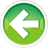# 1.9 — Introduction to expressions

Expressions

Consider the following series of statements:

Each of these statements defines a new variable and initializes it with a value. Note that the initializers shown above make use of a variety of different constructs: literals, variables, and operators. Somehow, C++ is converting each of these literals, variables, and operators into a single value that can be used as the initialization value for the variable.

What do all of these have in common? They make use of an expression.

An expression is a combination of literals, variables, operators, and explicit function calls (not shown above) that produce a single output value. When an expression is executed, each of the terms in the expression is evaluated until a single value remains (this process is called evaluation). That single value is the result of the expression.

Here are some examples of different kinds of expressions, with comments indicating how they evaluate:

As you can see, literals evaluate to their own values. Variables evaluate to the value of the variable. We haven’t covered function calls yet, but in the context of an expression, function calls evaluate whatever value the function returns. And operators let us combine multiple values together to produce a new value.

Note that expressions do not end in a semicolon, and cannot be compiled by themselves. For example, if you were to try compiling the expression x = 5, your compiler would complain (probably about a missing semicolon). Rather, expressions are always evaluated as part of statements.

For example, take this statement:

If you were to break this statement down into its syntax, it would look like this:

Type could be any valid type (we chose int). Identifier could be any valid name (we chose x). And expression could be any valid expression (we chose 2 + 3, which uses 2 literals and an operator).

Key insight

Wherever you can use a single value in C++, you can use an expression instead, and the compiler will resolve the expression down to a single value.

Expression statements

Certain expressions (like x = 5) are useful by themselves. However, we mentioned above that expressions can’t be compiled, so how can we use these expressions by themselves?

Fortunately, we can convert any expression into an equivalent statement. An expression statement is a statement that consists of an expression followed by a semicolon. This will create a statement that will execute just that expression.

Thus, we can take any expression (such as x = 5), and turn it into an expression statement (such as x = 5;) that will compile.

Note that we can make expression statements that compile but are meaningless/useless (e.g. 2;). This expression evaluates to 2, and then the value 2 is discarded.

Rule

Values calculated in an expression are discarded at the end of the expression.

Quiz time

Question #1

What is the difference between a statement and an expression?

Show Solution

Question #2

Indicate whether each of the following lines are statements that do not contain expressions, statements that contain expressions, or are expression statements.

a)

Show Solution

b)

Show Solution

c)

Show Solution

d) Extra credit:

Show Solution

Question #3

Determine what values the following program outputs. Do not compile this program. Just work through it line by line in your head.

Show Solution1.10 -- Developing your first programIndex1.8 -- Introduction to literals and operators

### 42 comments to 1.9 — Introduction to expressions

•nascardriver

test